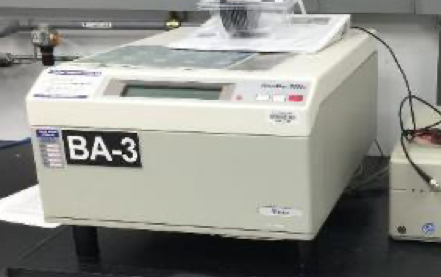# KLA Tencor Omnimapper 4 Point Probe

Description

The Tencor Omnimapper measures the sheet resistance of thin conductive and semiconductive films grown on a flat surface, usually a wafer. The Omnimapper is a 4 point probe where each probe needle is arranged in a line and spaced approximately 1 mm apart. The needles are part of a mechanical stage which moves up and down so that the needles contact the sample surface. A high impedance current source is passed through the two outer probes and a voltmeter measures the voltage across the two inner probes to calculate the resistivity. Using this setup and assuming that the tip is infinitesimal while the sample is semi infinite in the non-vertical direction, the sheet resistance (Rs) can be expressed as = Rs= πln2 VI where V is the voltage measured between the two inner probes and I is the current supplied to the outer two probes. Once the sheet resistance is extracted, the resistivity can be calculated by multiplying the sheet resistance by the film thickness.

Techniques
• 4 point probe
ASU Unit
Knowledge Enterprise
Rates
Service ASU rate Nonprofit/other academic rate Notes
Tool Usage \$33/hr \$43/hr
Photos